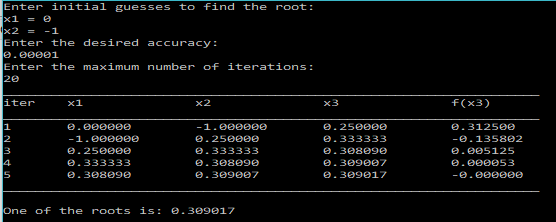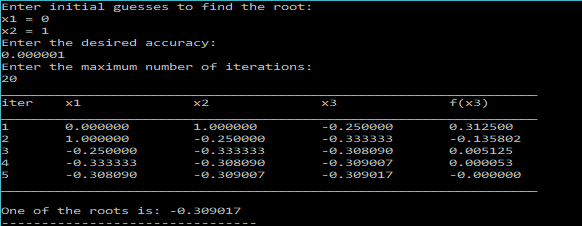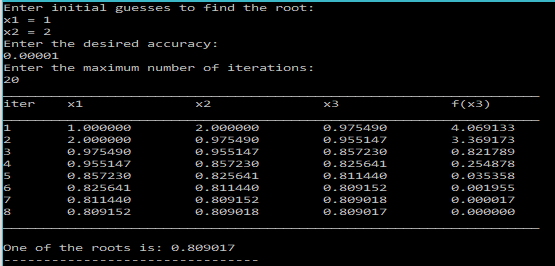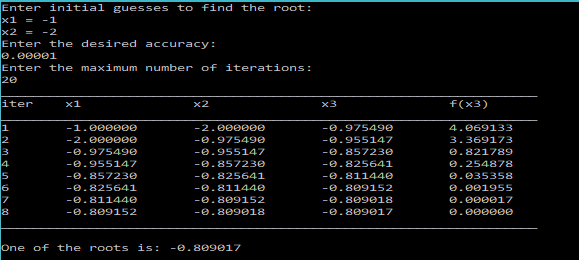# Numerical Root Finding Exercise – C Programs

In the last post, I showed you guys how to calculate and plot the Chebyshev Polynomials of the second kind.
And, in the second last post I showed you guys how to find the roots of an equation using the Secant Method.

### Exercise:

So, in this post, we would use the Secant method to find the roots of the Chebyshev Polynomial$U_4(x)$ of the second kind in the range [-1,1].

We would also plot it before finding the roots.

### Solution:

I won’t explain much about the calculating Chebyshev Polynomials, or the Secant Method as I have already wrote about them in detail in their specific posts. You can go ahead and check them out, before reading this one.

So in this program, we would be reusing most of our previous code for Chebyshev Polynomials and we would create one more function `U4(x)` specifically for the$U_4(x)$ as our problem demands. We would also reuse the function `secantPrint(...)`, that will calculate and return the root based on the initial guesses given and also tabulate the iterations.

### PROGRAM:

```/*************************************************
*******ROOT FINDING EXERCISE-PROBLEM 4.6.5********
Plot and find the roots of the Chebyshev polynomial of
the II kind U4(x) in the range [-1,1]*/
#include<stdio.h>
#include<math.h>
double U0(double x){
return 1;
}
double U1(double x){
return 2*x;
}
//General form of Chebyshev polynomial of second for a given value of n and x
double Un(double x, int n){
if(n==0){
return U0(x);
}
else if(n==1){
return U1(x);
}
else{
//using the recurrence relation
return 2*x*Un(x,n-1)-Un(x,n-2);
}
}
//function for U4(x)
double U4(double x){
return Un(x,4);
}
/*Secant Method Function that tabulates the values at each iteration*/
double secantPrint(double f(double x), double x1, double x2, double eps, int maxSteps){
int iter=1;
double x3;
printf("___________________________________________________________________\n");
printf("iter\tx1\t\tx2\t\tx3\t\tf(x3)\n");
printf("___________________________________________________________________\n");
do{
x3=(x1*f(x2)-x2*f(x1))/(f(x2)-f(x1));
printf("%d\t%lf\t%lf\t%lf\t%lf\n",iter,x1,x2,x3,f(x3));
x1=x2;
x2=x3;
iter++;
}while(fabs(f(x3))>eps&&iter<=maxSteps);
printf("___________________________________________________________________\n");
return x3;
}
main(){
double x,x1,x2,root,eps;
int maxSteps;
FILE *fp=NULL;
fp=fopen("chebyU4.txt","w");
//Write down the values to a file for plotting
for(x=-1;x<=1;x=x+0.01){
fprintf(fp,"%lf\t%lf\n",x,Un(x,4));
}
printf("Enter initial guesses to find the root:\nx1 = ");
scanf("%lf",&x1);
printf("x2 = ");
scanf("%lf",&x2);
printf("Enter the desired accuracy:\n");
scanf("%lf",&eps);
printf("Enter the maximum number of iterations:\n");
scanf("%d",&maxSteps);
root=secantPrint(U4,x1,x2,eps,maxSteps);
printf("\nOne of the roots is: %lf",root);
}
```

When you run, the above program, it will first create a file called `'chebyU4.txt'` that will contain the data-points for$U_4(x)$.
We can plot these using GnuPlot.

### Gnuplot Command

`plot 'chebyU4.txt' w l`

### OUTPUT(Gnuplot):### OUTPUT(C):

When, you run the above program, after writing the data-points to the text file(almost instantly), it will prompt you to enter the initial guesses, accuracy and max iterations for the Secant Method. Now since Secant Method returns a different root depending on the initial guesses, you will have to run the program a few times to find all the roots.
Based on the plot we just generated, we can see that there are 4 roots, that is, the function crosses the x-axis at 4-points.

Outputs based on different initial guesses are shown below.We can see that all of them are different, hence we have found the 4 roots of the Chebyshev polynomial$U_4(x)$.

### Verification:

We can verify our results by analytically calculating the roots of$U_4(x)$:$U_4(x)=16x^4-12x^2+1$
which is a degree 4 polynomial.

The roots are:
0.809 ; -0.809 ; 0.309 ; -.309.

### References:

http://mathworld.wolfram.com/ChebyshevPolynomialoftheSecondKind.html[wpedon id="7041" align="center"]